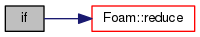The OpenFOAM Foundation
temperatureAndPressureVariables.H File Reference

Provides accumulation variables for temperatureAndPressure.H. More...

Go to the source code of this file.

## Functions

vector accumulatedTotalLinearMomentum (Zero)

if (Pstream::parRun())

## Variables

scalar accumulatedTotalMass = 0.0

scalar accumulatedTotalAngularKE = 0.0

scalar accumulatedTotalLinearKE = 0.0

scalar accumulatedTotalPE = 0.0

scalar accumulatedTotalrDotfSum = 0.0

label accumulatedNMols = 0

label accumulatedDOFs = 0

scalar averageTemperature = 0.0

scalar averagePressure = 0.0

const scalarFieldcellVols = mesh.cellVolumes()

scalar meshVolume = sum(cellVols)

## Detailed Description

Provides accumulation variables for temperatureAndPressure.H.

Original source file temperatureAndPressureVariables.H

Definition in file temperatureAndPressureVariables.H.

## Function Documentation

 vector accumulatedTotalLinearMomentum ( Zero )
 if ( Pstream::parRun() )

Definition at line 53 of file temperatureAndPressureVariables.H.

References Foam::reduce().

Here is the call graph for this function:## Variable Documentation

 scalar accumulatedTotalMass = 0.0

Definition at line 31 of file temperatureAndPressureVariables.H.

 scalar accumulatedTotalAngularKE = 0.0

Definition at line 33 of file temperatureAndPressureVariables.H.

 scalar accumulatedTotalLinearKE = 0.0

Definition at line 35 of file temperatureAndPressureVariables.H.

 scalar accumulatedTotalPE = 0.0

Definition at line 37 of file temperatureAndPressureVariables.H.

 scalar accumulatedTotalrDotfSum = 0.0

Definition at line 39 of file temperatureAndPressureVariables.H.

 label accumulatedNMols = 0

Definition at line 41 of file temperatureAndPressureVariables.H.

 label accumulatedDOFs = 0

Definition at line 43 of file temperatureAndPressureVariables.H.

 scalar averageTemperature = 0.0

Definition at line 45 of file temperatureAndPressureVariables.H.

Referenced by if().

 scalar averagePressure = 0.0

Definition at line 47 of file temperatureAndPressureVariables.H.

Referenced by if().

 const scalarField& cellVols = mesh.cellVolumes()
 scalar meshVolume = sum(cellVols)

Definition at line 51 of file temperatureAndPressureVariables.H.

Referenced by if().